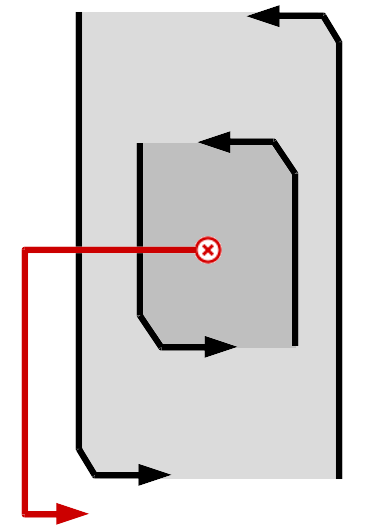# Programming-Idioms#### History of Idiom 43 > diff from v43 to v44

Edit summary for version 44 :
[C] Clarification of the previous comment (see edit)

#### Version 43

2019-09-26, 16:45:00

#### Version 44

2019-09-26, 17:31:27

# Idiom #43 Break outer loop

Look for a negative value v in 2D integer matrix m. Print it and stop searching.# Idiom #43 Break outer loop

Look for a negative value v in 2D integer matrix m. Print it and stop searching.##### Imports
`#include <stdio.h>`
##### Imports
`#include <stdio.h>`
##### Code
```int i,j;
for(i=0;i<sizeof(m)/sizeof(*m);i++)
{
for(j=0;j<sizeof(*m)/sizeof(**m);j++)
{
if(m[i][j]<0)
{
printf("%d\n",m[i][j]);
goto end;
}
}
}
end:```
##### Code
```int i,j;
for(i=0;i<sizeof(m)/sizeof(*m);i++)
{
for(j=0;j<sizeof(*m)/sizeof(**m);j++)
{
if(m[i][j]<0)
{
printf("%d\n",m[i][j]);
goto end;
}
}
}
end:```